Do you like the article?
Share it with others -
Use new possibilities of MetaTrader 5

4 January 2021, 08:24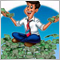1
3 161

### Introduction

In previous articles, we have discussed some types of neural network implementations. As you can see, neural networks are built of a large number of same type neurons, in which the same operations are performed. However, the more neurons a network has, the more computing resources it consumes. As a result, the time required to train a neural network grows exponentially, since the addition of one neuron to the hidden layer requires learning of connections with all neurons in the previous and next layers. There is a way to reduce the neural network training time. The multithreading capabilities of modern computers enable the calculation of multiple neurons simultaneously. Time will be considerably reduced due to an increase in the number of threads.

### 1. How multithreaded computing is organized in MQL5

However, there is a solution. The MetaTrader 5 developers have provided the ability to use third-party DLLs. Creation of dynamic libraries on a multithreaded architecture automatically provides multithreading of operations implemented in the library. Here, the EA operation along with the data exchange with the library remain in the main thread of the Expert Advisor.

The second option is to use the OpenCL technology. In this case, we can use standard means to organize multithreaded computing both on the processor supported by the technology and on video cards. For this option, the program code does not depend on the device utilized. There are a lot of publications related to the OpenCL technology on this site. In particular, the topic is well covered in articles  and .

So, I decided to use OpenCL. Firstly, when using this technology, users do not need to additionally configure the terminal and to set a permission to use third-party DLLs. Secondly, such an Expert Advisor can be transferred between terminals with one EX5 file. This allows the transfer of the calculation part to a video card, which capabilities are often idle during the operation of the terminal.

### 2. Multithreaded computing in neural networks

We have selected the technology. Now, we need to decide on the process of splitting calculations into threads. Do you remember the fully connected perceptron algorithm during a feed-forward pass? The signal moves sequentially from the input layer to hidden layers and then to the output layer. There is no point in allocating a thread for each layer, as calculations must be performed sequentially. A layer calculation cannot start until the result from the previous layer is received. The calculation of an individual neuron in a layer does not depend on the results of calculation of other neurons in this layer. It means that we can allocate separate threads for each neuron and send all neurons of a layer for parallel computation.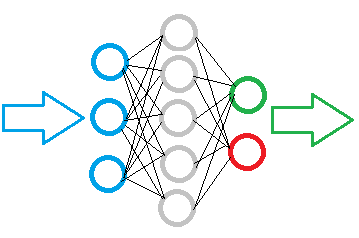Going down to the operations of one neuron, we could consider the possibility of parallelizing the calculation of the products of input values by their weight coefficients. However, further summation of the resulting values and the calculation of the activation function value are combined into a single thread. I decided to implement these operations in a single OpenCL kernel using vector functions.

A similar approach is used for splitting feed-backward threads. The implementation is shown below.

### 3. Implementing multithreaded computing with OpenCL

Having chosen the basic approaches, we can proceed to the implementation. Let us start with the creation of kernels (executable OpenCL functions). According to the above logic, we will create 4 kernels.

#### 3.1. Feed-forward kernel.

Similar to the methods discussed in previous articles, let us create a feed-forward pass kernel FeedForward.

Do not forget that the kernel is a function that runs in each thread. The number of such threads is set when calling the kernel. Operations inside the kernel are nested operations inside a certain loop; the number of iterations of the loop is equal to the number of the called threads. So, in the feed-forward kernel we can specify the operations for calculating a separate neuron state, and the number of neurons can be specified when calling the kernel from the main program.

The kernel receives in parameters references to the matrix of weights, an array of input data and an array of output data, as well as the number of elements of the input array and the activation function type. Pay attention that all arrays in OpenCL are one-dimensional. Therefore, if a two-dimensional array is used for weight coefficients in MQL5, here we need to calculate the shifts of the initial position in order to read the data of the second and subsequent neurons.

```__kernel void FeedForward(__global double *matrix_w,
__global double *matrix_i,
__global double *matrix_o,
int inputs, int activation)

```

At the beginning of the kernel, we get the sequence number of the thread which determines the sequence number of the calculated neuron. Declare private (internal) variables, including vector variables inp and weight. Also define the shift to the weights of our neuron.

```  {
int i=get_global_id(0);
double sum=0.0;
double4 inp, weight;
int shift=(inputs+1)*i;

```

Next, organize a cycle to obtain the sum of products of incoming values with their weights. As mentioned above, we used vectors of 4 elements inp and weight to calculate the sum of the products. However, not all arrays received by the kernel will be multiples of 4, so the missing elements should be replaced with zero values. Pay attention to one "1" in the input data vector - it will correspond to a weight of the Bayesian bias.

```   for(int k=0; k<=inputs; k=k+4)
{
switch(inputs-k)
{
case 0:
inp=(double4)(1,0,0,0);
weight=(double4)(matrix_w[shift+k],0,0,0);
break;
case 1:
inp=(double4)(matrix_i[k],1,0,0);
weight=(double4)(matrix_w[shift+k],matrix_w[shift+k+1],0,0);
break;
case 2:
inp=(double4)(matrix_i[k],matrix_i[k+1],1,0);
weight=(double4)(matrix_w[shift+k],matrix_w[shift+k+1],matrix_w[shift+k+2],0);
break;
case 3:
inp=(double4)(matrix_i[k],matrix_i[k+1],matrix_i[k+2],1);
weight=(double4)(matrix_w[shift+k],matrix_w[shift+k+1],matrix_w[shift+k+2],matrix_w[shift+k+3]);
break;
default:
inp=(double4)(matrix_i[k],matrix_i[k+1],matrix_i[k+2],matrix_i[k+3]);
weight=(double4)(matrix_w[shift+k],matrix_w[shift+k+1],matrix_w[shift+k+2],matrix_w[shift+k+3]);
break;
}
sum+=dot(inp,weight);
}

```

After obtaining the sum of the products, calculate the activation function and write the result into the output data array.

```   switch(activation)
{
case 0:
sum=tanh(sum);
break;
case 1:
sum=pow((1+exp(-sum)),-1);
break;
}
matrix_o[i]=sum;
}
```

#### 3.2. Backpropagation kernels.

Create two kernels to back propagate the error gradient. Calculate the output layer error in the first CaclOutputGradient. Its logic is simple. The obtained reference values are normalized within the values of the activation function. Then, the difference between the reference and actual values is multiplied by the derivative of the activation function. Write the resulting value into the corresponding cell of the gradient array.

```__kernel void CaclOutputGradient(__global double *matrix_t,
__global double *matrix_o,
__global double *matrix_ig,
int activation)
{
int i=get_global_id(0);
double temp=0;
double out=matrix_o[i];
switch(activation)
{
case 0:
temp=clamp(matrix_t[i],-1.0,1.0)-out;
temp=temp*(1+out)*(1-(out==1 ? 0.99 : out));
break;
case 1:
temp=clamp(matrix_t[i],0.0,1.0)-out;
temp=temp*(out==0 ? 0.01 : out)*(1-(out==1 ? 0.99 : out));
break;
}
matrix_ig[i]=temp;
}

```

In the second kernel, calculate the error gradient of the hidden layer neuron CaclHiddenGradient. The kernel building is similar to the feed-forward kernel described above. It also uses vector operations. The differences are in the use of the next layer gradient vector instead of the previous layer output values in the feed-forward pass and in the use of a different weight matrix. Also, instead of calculating the activation function, the resulting sum is multiplied by the derivative of the activation function. The kernel code is given below.

```__kernel void CaclHiddenGradient(__global double *matrix_w,
__global double *matrix_g,
__global double *matrix_o,
__global double *matrix_ig,
int outputs, int activation)
{
int i=get_global_id(0);
double sum=0;
double out=matrix_o[i];
int shift=(outputs+1)*i;
for(int k=0;k<outputs;k+=4)
{
switch(outputs-k)
{
case 0:
weight=(double4)(matrix_w[shift+k],0,0,0);
break;
case 1:
weight=(double4)(matrix_w[shift+k],matrix_w[shift+k+1],0,0);
break;
case 2:
weight=(double4)(matrix_w[shift+k],matrix_w[shift+k+1],matrix_w[shift+k+2],0);
break;
case 3:
weight=(double4)(matrix_w[shift+k],matrix_w[shift+k+1],matrix_w[shift+k+2],matrix_w[shift+k+3]);
break;
default:
weight=(double4)(matrix_w[shift+k],matrix_w[shift+k+1],matrix_w[shift+k+2],matrix_w[shift+k+3]);
break;
}
}
switch(activation)
{
case 0:
sum=clamp(sum+out,-1.0,1.0);
sum=(sum-out)*(1+out)*(1-(out==1 ? 0.99 : out));
break;
case 1:
sum=clamp(sum+out,0.0,1.0);
sum=(sum-out)*(out==0 ? 0.01 : out)*(1-(out==1 ? 0.99 : out));
break;
}
matrix_ig[i]=sum;
}

```

#### 3.3. Updating the weights.

Let us create another kernel for updating the weights - UpdateWeights. The procedure for updating each individual weight does not depend on other weights within one neuron and from other neurons. This allows the sending of tasks for parallel computation of all weights of all neurons in one layer at the same time. In this case, we run one kernel in a two-dimensional space of threads: one dimension indicates the serial number of the neuron, and the second dimension means the number of connections within the neuron. This is shown in the first 2 lines of the kernel code, where it receives thread IDs in two dimensions.

```__kernel void UpdateWeights(__global double *matrix_w,
__global double *matrix_g,
__global double *matrix_i,
__global double *matrix_dw,
int inputs, double learning_rates, double momentum)
{
int i=get_global_id(0);
int j=get_global_id(1);
int wi=i*(inputs+1)+j;
double delta=learning_rates*matrix_g[i]*(j<inputs ? matrix_i[j] : 1) + momentum*matrix_dw[wi];
matrix_dw[wi]=delta;
matrix_w[wi]+=delta;
};

```

Next, determine the shift for the updated weight in the array of weights, calculate the delta (change), then add the resulting value into the array of deltas and add it to the current weight.

All kernels are placed in a separate file NeuroNet.cl, which will be connect as a resource to the main program.

`#resource "NeuroNet.cl" as string cl_program`

#### 3.4. Creating classes of the main program.

After creating kernels, let us get back to MQL5 and start working with the main program code. Data between the main program and the kernels is exchanged through buffers of one-dimensional arrays (this is explained in article ). To organize such buffers on the main program side, let us create the CBufferDouble class. This class contains a reference to the object of the class for working with OpenCL and the index of the buffer which it receives when created in OpenCL.

```class CBufferDouble     :  public CArrayDouble
{
protected:
COpenCLMy         *OpenCL;
int               m_myIndex;
public:
CBufferDouble(void);
~CBufferDouble(void);
//---
virtual bool      BufferInit(uint count, double value);
virtual bool      BufferCreate(COpenCLMy *opencl);
virtual bool      BufferFree(void);
virtual bool      BufferWrite(void);
virtual int       GetData(double &values[]);
virtual int       GetData(CArrayDouble *values);
virtual int       GetIndex(void)                        {  return m_myIndex;      }
//---
virtual int       Type(void)                      const { return defBufferDouble; }
};
```

Pay attention that upon the creation of the OpenCL buffer its handle is returned. This handle is stored in the m_buffers array of the COpenCL class. In the m_myIndex variable only the index in the specified array is stored. This is because the entire COpenCL class operation uses the specification of such an index, not the kernel or buffer handle. Also note that the COpenCL class operation algorithm out of the box requires the initial specification of the number of used buffers and further creation of buffers with a specific index. In our case, we will dynamically add buffers when creating neural layers. That is why the COpenCLMy class is derived from COpenCL. This class contains only one additional method. You can find its code in the attachment.

The following methods have been created in the CBufferDouble class for working with the buffer:

• BufferInit — buffer array initialization with the specified value
• BufferCreate  — create a buffer in OpenCL
• BufferFree  — delete a buffer in OpenCL
• BufferRead  — read data from the OpenCL buffer to an array
• BufferWrite  — write data from the array to the OpenCL buffer
• GetData  — get array data on request. It is implemented in two variants to return data to an array and CArrayDouble class
• GetIndex  — returns the buffer index

The architecture of all methods is quite simple and their code takes in 1-2 lines. The full code of all methods is provided in the attachment below.

#### 3.5. Creating a base neuron class for working with OpenCL.

Let us move on and consider the CNeuronBaseOCL class which includes the main additions and operation algorithm. It is difficult to name the created object a neuron, since it contains the work of the entire fully connected neural layer. The same applies to the earlier considered convolutional layers and LSTM blocks. But this approach allows preserving of the previously built neural network architecture.

Class CNeuronBaseOCL contains a pointer to the COpenCLMy class object and four buffers: output values, a matrix of weight coefficients, last weight deltas and error gradients.

```class CNeuronBaseOCL    :  public CObject
{
protected:
COpenCLMy         *OpenCL;
CBufferDouble     *Output;
CBufferDouble     *Weights;
CBufferDouble     *DeltaWeights;
```

Also, declare the learning and momentum coefficient, the ordinal number of the neuron in the layer and the activation function type.

```   const double      eta;
const double      alpha;
//---
int               m_myIndex;
ENUM_ACTIVATION   activation;

```

Add three more methods to the protected block: feed-forward, hidden gradient calculation and update of the weight matrix.

```   virtual bool      feedForward(CNeuronBaseOCL *NeuronOCL);
virtual bool      updateInputWeights(CNeuronBaseOCL *NeuronOCL);

```

In the public block, declare class constructor and destructor, neuron initialization method and a method for specifying the activation function.

```public:
CNeuronBaseOCL(void);
~CNeuronBaseOCL(void);
virtual bool      Init(uint numOutputs, uint myIndex, COpenCLMy *open_cl, uint numNeurons);
virtual void      SetActivationFunction(ENUM_ACTIVATION value) {  activation=value; }

```

For external access to data from neurons, declare methods for obtaining buffer indices (they will be used when calling kernels) and methods for receiving current information from buffers in the form of arrays. Also, add methods for polling the number of neurons and activation functions.

```   virtual int       getOutputIndex(void)          {  return Output.GetIndex();        }
virtual int       getWeightsIndex(void)         {  return Weights.GetIndex();       }
virtual int       getDeltaWeightsIndex(void)    {  return DeltaWeights.GetIndex();  }
//---
virtual int       getOutputVal(double &values[])   {  return Output.GetData(values);      }
virtual int       getOutputVal(CArrayDouble *values)   {  return Output.GetData(values);  }
virtual int       getWeights(double &values[])     {  return Weights.GetData(values);     }
virtual int       Neurons(void)                    {  return Output.Total();              }
virtual ENUM_ACTIVATION Activation(void)           {  return activation;                  }

```

And, of course, create dispatching methods for feed-forward pass, error gradient calculation and updating of the weight matrix. Do not forget to rewrite the virtual functions for saving and reading data.

```   virtual bool      feedForward(CObject *SourceObject);
virtual bool      updateInputWeights(CObject *SourceObject);
//---
virtual bool      Save(int const file_handle);
//---
virtual int       Type(void)        const                      {  return defNeuronBaseOCL;                  }
};

```

Let us consider the algorithms for constructing methods. Class constructor and destructor are rather simple. Their code is available in the attachment. Take a look at the class initialization function. The method receives in parameters the number of neurons in the next layer, the ordinal number of the neuron, a pointer to the COpenCLMy class object and the number of neurons to be created.

Note that the method receives in parameters a pointer to the COpenCLMy class object and it does not instantiate an object inside the class. This ensures that only one instance of the COpenCLMy object is used during EA operation. All kernels and data buffers will be created in one object, so we will not need to waste time passing data between the layers of the neural network. They will have direct access to the same data buffers.

At the method beginning, check the validity of the pointer to the COpenCLMy class object and make sure that at least one neuron should be created. Next, create instances of buffer objects, initialize arrays with initial values and create buffers in OpenCL. The size of the 'Output' buffer is equal to the number of neurons to be created and the size of the gradients buffer is 1 element larger. The sizes of weight matrix and their delta buffers are equal to the product of the gradients buffer size by the number of neurons in the next layer. Since this product will be "0" for the output layer, buffers are not created for this layer.

```bool CNeuronBaseOCL::Init(uint numOutputs,uint myIndex,COpenCLMy *open_cl,uint numNeurons)
{
if(CheckPointer(open_cl)==POINTER_INVALID || numNeurons<=0)
return false;
OpenCL=open_cl;
//---
if(CheckPointer(Output)==POINTER_INVALID)
{
Output=new CBufferDouble();
if(CheckPointer(Output)==POINTER_INVALID)
return false;
}
if(!Output.BufferInit(numNeurons,1.0))
return false;
if(!Output.BufferCreate(OpenCL))
return false;
//---
{
return false;
}
return false;
return false;
//---
if(numOutputs>0)
{
if(CheckPointer(Weights)==POINTER_INVALID)
{
Weights=new CBufferDouble();
if(CheckPointer(Weights)==POINTER_INVALID)
return false;
}
int count=(int)((numNeurons+1)*numOutputs);
if(!Weights.Reserve(count))
return false;
for(int i=0;i<count;i++)
{
double weigh=(MathRand()+1)/32768.0-0.5;
if(weigh==0)
weigh=0.001;
return false;
}
if(!Weights.BufferCreate(OpenCL))
return false;
//---
if(CheckPointer(DeltaWeights)==POINTER_INVALID)
{
DeltaWeights=new CBufferDouble();
if(CheckPointer(DeltaWeights)==POINTER_INVALID)
return false;
}
if(!DeltaWeights.BufferInit(count,0))
return false;
if(!DeltaWeights.BufferCreate(OpenCL))
return false;
}
//---
return true;
}

```

The feedForward dispatcher method is similar to the method of the same name of the CNeuronBase class. Now, only one type of neurons is specified here, but more types can be added later.

```bool CNeuronBaseOCL::feedForward(CObject *SourceObject)
{
if(CheckPointer(SourceObject)==POINTER_INVALID)
return false;
//---
CNeuronBaseOCL *temp=NULL;
switch(SourceObject.Type())
{
case defNeuronBaseOCL:
temp=SourceObject;
return feedForward(temp);
break;
}
//---
return false;
}
```

The OpenCL kernel is called directly in the feedForward(CNeuronBaseOCL *NeuronOCL) method. At the method beginning, check the validity of the pointer to the COpenCLMy class object and of the received pointer to the previous layer of the neural network.

```bool CNeuronBaseOCL::feedForward(CNeuronBaseOCL *NeuronOCL)
{
if(CheckPointer(OpenCL)==POINTER_INVALID || CheckPointer(NeuronOCL)==POINTER_INVALID)
return false;

```

Indicate the one-dimensionality of the treads space and set the number of required threads equal to the number of neurons.

```   uint global_work_offset={0};
uint global_work_size;
global_work_size=Output.Total();
```

Next, set pointers to the used data buffers and arguments for the kernel operation.

```   OpenCL.SetArgumentBuffer(def_k_FeedForward,def_k_ff_matrix_w,NeuronOCL.getWeightsIndex());
OpenCL.SetArgumentBuffer(def_k_FeedForward,def_k_ff_matrix_i,NeuronOCL.getOutputIndex());
OpenCL.SetArgumentBuffer(def_k_FeedForward,def_k_ff_matrix_o,Output.GetIndex());
OpenCL.SetArgument(def_k_FeedForward,def_k_ff_inputs,NeuronOCL.Neurons());
OpenCL.SetArgument(def_k_FeedForward,def_k_ff_activation,(int)activation);

```

After that call the kernel.

```   if(!OpenCL.Execute(def_k_FeedForward,1,global_work_offset,global_work_size))
return false;

```

I wanted to finish here, but I ran into a problem during testing: the COpenCL::Execute method does not launch the kernel, but only queues it. The execution itself occurs at the attempt to read the results of the kernel. That is why the processing results have to be loaded into an array before exiting the method.

```   Output.BufferRead();
//---
return true;
}

```

Methods for launching other kernels are similar to the above algorithm. The full code of all methods and classes is available in the attachment.

#### 3.6. Additions in CNet class.

Once all the necessary classes have been created, let us make some adjustments to the CNet class of the main neural network.

In the class constructor, we need to add the creation and initialization of an COpenCLMy class instance. Do not forget to delete the class object in the destructor.

```   opencl=new COpenCLMy();
if(CheckPointer(opencl)!=POINTER_INVALID && !opencl.Initialize(cl_program,true))
delete opencl;

```

Also, in the constructor, in the block adding neurons in layers, add a code creating and initializing objects of the earlier created CNeuronBaseOCL class.

```      if(CheckPointer(opencl)!=POINTER_INVALID)
{
CNeuronBaseOCL *neuron_ocl=NULL;
switch(desc.type)
{
case defNeuron:
case defNeuronBaseOCL:
neuron_ocl=new CNeuronBaseOCL();
if(CheckPointer(neuron_ocl)==POINTER_INVALID)
{
delete temp;
return;
}
if(!neuron_ocl.Init(outputs,0,opencl,desc.count))
{
delete temp;
return;
}
neuron_ocl.SetActivationFunction(desc.activation);
{
delete neuron_ocl;
delete temp;
return;
}
neuron_ocl=NULL;
break;
default:
return;
break;
}
}

```

Further, add the creation of kernels in OpenCL in the constructor.

```   if(CheckPointer(opencl)==POINTER_INVALID)
return;
//--- create kernels
opencl.SetKernelsCount(4);
opencl.KernelCreate(def_k_FeedForward,"FeedForward");
opencl.KernelCreate(def_k_UpdateWeights,"UpdateWeights");

```

Add writing of source data to buffer in the CNet::feedForward method

```     {
CNeuronBaseOCL *neuron_ocl=current.At(0);
double array[];
int total_data=inputVals.Total();
if(ArrayResize(array,total_data)<0)
return false;
for(int d=0;d<total_data;d++)
array[d]=inputVals.At(d);
if(!opencl.BufferWrite(neuron_ocl.getOutputIndex(),array,0,0,total_data))
return false;
}

```

Also add the appropriate method call of the newly created class CNeuronBaseOCL.

```   for(int l=1; l<layers.Total(); l++)
{
previous=current;
current=layers.At(l);
if(CheckPointer(current)==POINTER_INVALID)
return false;
//---
if(CheckPointer(opencl)!=POINTER_INVALID)
{
CNeuronBaseOCL *current_ocl=current.At(0);
if(!current_ocl.feedForward(previous.At(0)))
return false;
continue;
}

```

For the back-propagation process, let us create a new method CNet::backPropOCL. Its algorithm is similar to the main method CNet::backProp, which was described in the first article.

```void CNet::backPropOCL(CArrayDouble *targetVals)
{
if(CheckPointer(targetVals)==POINTER_INVALID || CheckPointer(layers)==POINTER_INVALID || CheckPointer(opencl)==POINTER_INVALID)
return;
CLayer *currentLayer=layers.At(layers.Total()-1);
if(CheckPointer(currentLayer)==POINTER_INVALID)
return;
//---
double error=0.0;
int total=targetVals.Total();
double result[];
CNeuronBaseOCL *neuron=currentLayer.At(0);
if(neuron.getOutputVal(result)<total)
return;
for(int n=0; n<total && !IsStopped(); n++)
{
double target=targetVals.At(n);
double delta=(target>1 ? 1 : target<-1 ? -1 : target)-result[n];
error+=delta*delta;
}
error/= total;
error = sqrt(error);
recentAverageError+=(error-recentAverageError)/recentAverageSmoothingFactor;

return;;
CObject *temp=NULL;
total=layers.Total();
for(int layerNum=total-2; layerNum>0; layerNum--)
{
CLayer *nextLayer=currentLayer;
currentLayer=layers.At(layerNum);
neuron=currentLayer.At(0);
}
//---
CLayer *prevLayer=layers.At(total-1);
for(int layerNum=total-1; layerNum>0; layerNum--)
{
currentLayer=prevLayer;
prevLayer=layers.At(layerNum-1);
neuron=currentLayer.At(0);
neuron.updateInputWeights(prevLayer.At(0));
}
}

```

Some minor changes have been made to the getResult method.

```   if(CheckPointer(opencl)!=POINTER_INVALID && output.At(0).Type()==defNeuronBaseOCL)
{
CNeuronBaseOCL *temp=output.At(0);
temp.getOutputVal(resultVals);
return;
}

```

The full code of all methods and functions is available in the attachment.

### 4. Testing

The created class operation was tested under the same conditions that we used in previous tests. The Fractal_OCL EA has been created for testing, which is a complete analogue of the previously created Fractal_2. Test training of the neural network was carried out on the EURUSD pair, on the H1 timeframe. Data on 20 candlesticks was input into the neural network. Training was performed using data for the last 2 years. Experiment was performed on a CPU device 'Intel(R) Core(TM)2 Duo CPU T5750 @ 2.00GHz' with OpenCL support.

For 5 hours and 27 minutes of testing, the EA using the OpenCL technology executed 75 training epochs. This gave on average 4 minutes and 22 seconds for an epoch of 12,405 candles. The same Expert Advisor without OpenCL technology, on the same laptop with the same neural network architecture spends an average of 40 minutes 48 seconds per epoch. So, the learning process is 9.35 times faster with OpenCL.

### Conclusion

This article has demonstrated the possibility of using OpenCL technology for organizing multithreaded computations in neural networks. Testing has shown an almost 10-fold increase in performance on the same CPU. It is expected that the use of a GPU can further improve the algorithm performance - in this case, transferring of calculations to a compatible GPU does not require changes in the Expert Advisor code.

In general, the results prove that further development of this direction has good prospects.

### Programs used in the article

# Name Type Description
1 Fractal_OCL.mq5  Expert Advisor An Expert Advisor with the classification neural network (3 neurons in the output layer) using the OpenCL technology
2 NeuroNet.mqh Class library A library of classes for creating a neural network
3 NeuroNet.cl Code Base OpenCL program code library

Translated from Russian by MetaQuotes Software Corp.
Original article: https://www.mql5.com/ru/articles/8435

Attached files |
MQL5.zip (396.86 KB)
Last comments | Go to discussion (1)| 13 Jan 2021 at 12:44

Thank you for these articles! It's been very interesting following the ideas of this system.

I'm very interested in the LSTM-module, is there a way to use Open-CL for LSTM-Networks?

I've been trying to modify the LSTM-EA to work with Open-CL, but with no success.

Best regards

Pontus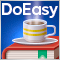Timeseries in DoEasy library (part 55): Indicator collection class

The article continues developing indicator object classes and their collections. For each indicator object create its description and correct collection class for error-free storage and getting indicator objects from the collection list.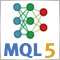Neural networks made easy (Part 4): Recurrent networks

We continue studying the world of neural networks. In this article, we will consider another type of neural networks, recurrent networks. This type is proposed for use with time series, which are represented in the MetaTrader 5 trading platform by price charts.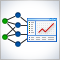Practical application of neural networks in trading. Python (Part I)

In this article, we will analyze the step-by-step implementation of a trading system based on the programming of deep neural networks in Python. This will be performed using the TensorFlow machine learning library developed by Google. We will also use the Keras library for describing neural networks.Timeseries in DoEasy library (part 56): Custom indicator object, get data from indicator objects in the collection

The article considers creation of the custom indicator object for the use in EAs. Let’s slightly improve library classes and add methods to get data from indicator objects in EAs.